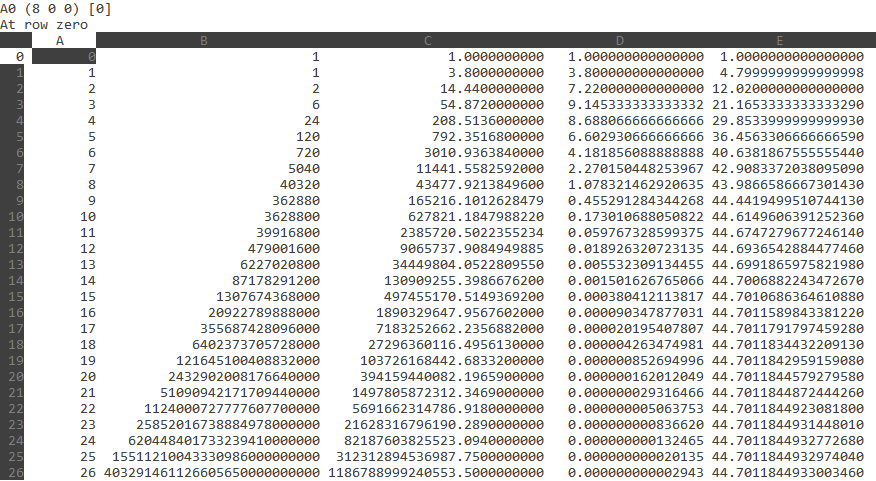# How do you evaluate e^3.8?

How do you evaluate e^3.8?

ReportAnswer 1

Use a calculator, or sum the series to find:

${e}^{3.8} \approx 44.70118449330082303755$

#### Explanation:

If your calculator has an ${e}^{x}$ function then you can use that to find:

${e}^{3.8} \approx 44.70118449330082303755$

Otherwise, you might like to try the standard series:

e^x = sum_(n=0)^oo x^n/(n!)

It takes a few terms to converge.

Here I evaluated it in a spreadsheet:Column A contains the value of $n$

Column B calculates n!

Column C calculates ${3.8}^{n}$

Column D calculates 3.8^n/(n!)

Column E sums the values from column D

##### Add Answer of: How do you evaluate e^3.8?
Similar Homework Help Questions

• ### How do you evaluate e^ (ln sqrt20)?

Need Online Homework Help?